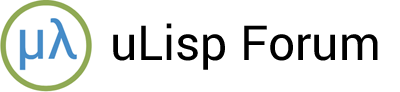# Common Lisp LAST function

#1

I’ve found it useful to have a subset of the Common Lisp LAST function. In particular I was interested in doing circular list to implement a port of https://rosettacode.org/wiki/Averages/Simple_moving_average#Common_Lisp to uLisp

``````(defun sma (qlist)
(let ((period (length qlist))
(sum (apply #'+ qlist))
(qptr qlist))
(setf (rest (last qlist)) qlist)
(lambda (val)
(decf sum (first qptr))
(incf sum val)
(setf (first qptr) val)
(setf qptr (rest qptr))
(/ sum period))))

(defvar test (sma '(4 4 4 4)))
(test 9)
``````

Since I need it to work with SETF, it required more than just a fn_last()…

If anyone would find it useful:

`````` object *fn_last (object *args, object *env) {
(void) env;
object *list = first(args);
if (list == NULL) return nil;
if (atom(list)) error(LAST, PSTR("first argument to last is not a list"), list);
while (list != NULL && listp(list)) {
object *tail = cdr(list);
if (atom(tail) || tail == NULL)  break;
list = tail;
}
return list;
}
``````

and in object **place()

``````if (fname == LAST) {
object *list = eval(second(args), env);
if (list == NULL) return nil;
if (atom(list)) error(LAST, PSTR("first argument to last is not a list"), list);
while (list != NULL && listp(list)) {
object *tail = cdr(list);
if (atom(tail) || tail == NULL)  break;
list = tail;
}
return &list;
}
``````

I’m sure this can be much improved (this was my first cut), but I am hoping that a subset implementation of LAST would be a worthwhile addition.